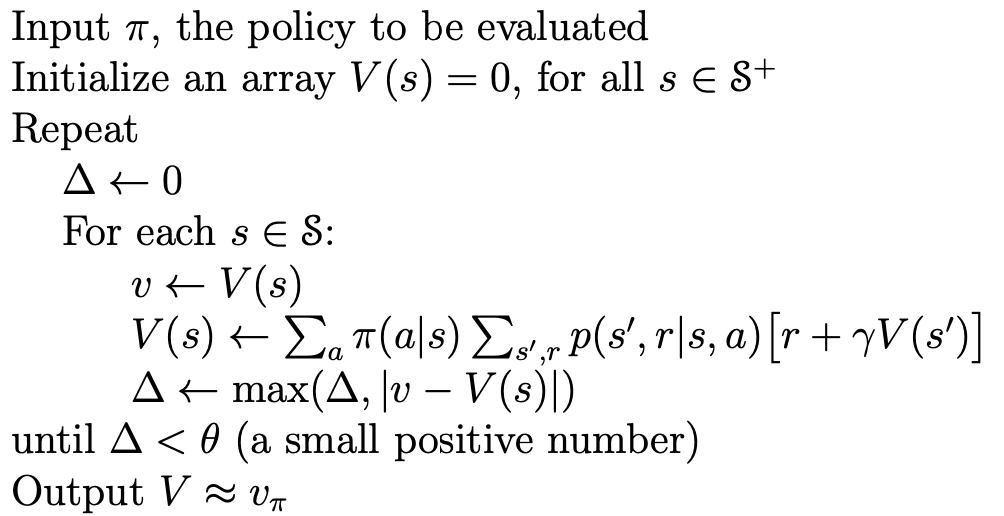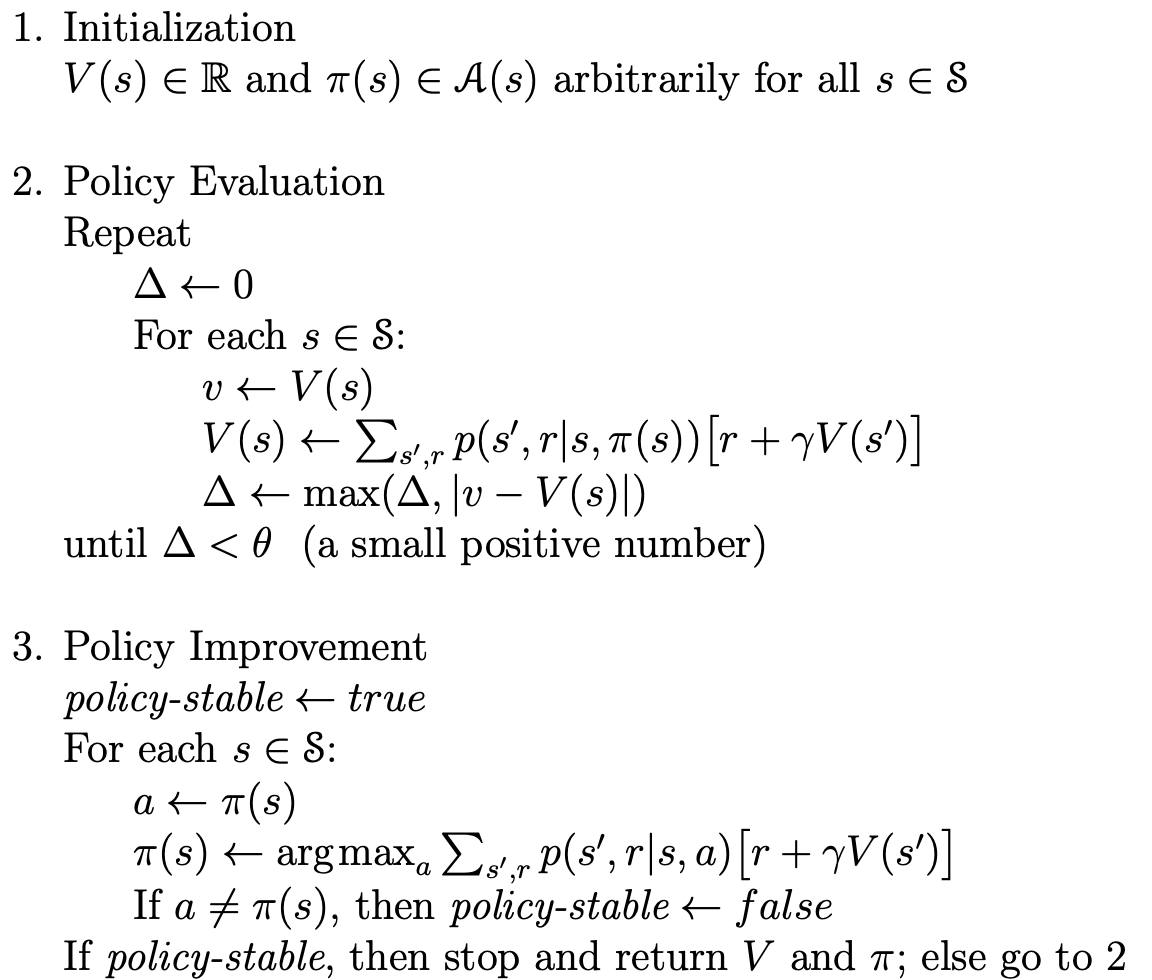# Dynamic Programming

​  Dynamic Programming 指的是可用于在给定环境的情况下计算最优策略的算法。经典的动态规划算法在强化学习中的作用非常有限，因为它通常需要一个非常完美的数学模型和巨大的计算开销，但是这些思想在设计强化学习的算法时还是非常重要的。 本章所要介绍的动态规划算法为理解本书其余部分的方法提供了重要的基础，事实上，所有这些方法都可以看作是动态规划算法的具有更小计算量的一个近似。

​  从本章开始，我们通常假设环境是一个有限的 MDP。也就是说，我们假设它的state、action和reward 集合 SA(s)R，对于 s \in S 是有限的，并且对于所有 s \in Sa \in A(s)r \in Rs′ \in S+，它的动态由一组概率 p(s′,r |s,a) 来描述。

​  Dynamic Programming 的关键思想是使用价值函数来搜索好的策略。在本章中，我们将展示如何使用 Dynamic Programming 来计算前面定义的价值函数。正如之前所讨论的，一旦我们找到满足贝尔曼最优性条件的最优价值函数 v^*q^*，我们就可以得到最优策略了。

\begin{aligned}
v_{*}(s) &=\max _{a} \mathbb{E}\left[R_{t+1}+\gamma v_{*}\left(S_{t+1}\right) \mid S_{t}=s, A_{t}=a\right] \\
&=\max _{a \in \mathcal{A}(s)} \sum_{s^{\prime}, r} p\left(s^{\prime}, r \mid s, a\right)\left[r+\gamma v_{*}\left(s^{\prime}\right)\right] .
\end{aligned}
\begin{aligned}
q_{*}(s, a) &=\mathbb{E}\left[R_{t+1}+\gamma \max _{a^{\prime}} q_{*}\left(S_{t+1}, a^{\prime}\right) \mid S_{t}=s, A_{t}=a\right] \\
&=\sum_{s^{\prime}, r} p\left(s^{\prime}, r \mid s, a\right)\left[r+\gamma \max _{a^{\prime}} q_{*}\left(s^{\prime}, a^{\prime}\right)\right]
\end{aligned}

​  下面我们就开始分析如何从任意策略出发获得最优策略，大体的思路是：先任意给出一个策略，然后对这个策略进行验证，也就是求得这个策略的值函数，这个步骤称作策略验证，继而对策略进行优化，然后对优化的策略再次求解值函数，直到获得最优策略，值得注意的是，每次优化完策略的时候对需要检验一下当前是不是最优策略，如果是的话则迭代可以停止。

## Policy Evaluation

​  验证一个策略指的是求出当前策略下的值函数，而初始的值函数往往是随机的，而我们又没有什么办法可以直接将这个值函数求解出来，因此这里采用一种迭代的方法将其求解出来。因此，在对策略的迭代中，连接相邻两次迭代的策略验证事实上是对一系列迭代的抽象。

​  首先，我们来看如何计算任意策略 \pi 的状态值函数 v_{\pi}，之前说过的状态 s 的值函数的迭代计算如下所示：

\begin{aligned}
v_{\pi}(s) &=\mathbb{E}_{\pi}\left[\sum_{k=0}^{\infty} \gamma^{k} R_{t+k+1} \mid S_{t}=s\right] \\
&=\mathbb{E}_{\pi}\left[R_{t+1}+\gamma \sum_{k=0}^{\infty} \gamma^{k} R_{t+k+2} \mid S_{t}=s\right]\\
&=\sum_{a} \pi(a \mid s) \sum_{s^{\prime}, r} p\left(s^{\prime}, r \mid s, a\right)\left[r+\gamma v_{\pi}\left(s^{\prime}\right)\right]
\end{aligned}

​  对于每个策略 \pi，迭代的具体过程如下所示：

\begin{aligned}
v_{k+1}(s) = \sum_{a} \pi(a \mid s) \sum_{s^{\prime}, r} p\left(s^{\prime}, r \mid s, a\right)\left[r+\gamma v_k\left(s^{\prime}\right)\right]
\end{aligned}

​  为了从 v_k 逐次逼近 v_{k+1}，策略验证对每个状态 s 应用相同的操作：它将 s 的值替换为从 s 的后继状态的值获得的新值，这种操作被称为 full\ backup。策略验证的每次迭代都会为每个状态的值进行一次备份，以生成新的近似 v_{k+1}。在 Dynamic Programming 算法中完成的所有备份都称为完全备份，因为它们基于所有可能的下一个状态。策略验证算法的伪代码如图所示：## Policy Improvement

​  策略提升是 Dynamic Programming 的关键，这一步就是在优化我们的策略了！我们计算策略的价值函数是为了找到更好的策略。假设我们已经确定了任意策略 \pi 的价值函数 v_{\pi}，对于某些状态 s，我们想知道我们是否应该改变策略来确定性地选择一个动作 a \neq \pi(s)。在完成了Policy Evaluation之后，我们可以知道当前的策略有多好，但是如何检验新策略会更好还是更糟糕？一种方法是考虑在 s 下选择 a，然后遵循现有策略 \pi 对动作 a 的值函数进行验证，并与策略 \pi 的值函数进行比较：

\begin{aligned}
q_{\pi}(s,a)&=\sum_{s^{\prime}, r} p\left(s^{\prime}, r \mid s, a\right)\left[r+\gamma v_{\pi}\left(s^{\prime}\right)\right]
\end{aligned}

\begin{aligned}
v_{\pi}(s) & \leq q_{\pi}\left(s, \pi^{\prime}(s)\right) \\
&=\mathbb{E}_{\pi^{\prime}}\left[R_{t+1}+\gamma v_{\pi}\left(S_{t+1}\right) \mid S_{t}=s\right] \\
& \leq \mathbb{E}_{\pi^{\prime}}\left[R_{t+1}+\gamma q_{\pi}\left(S_{t+1}, \pi^{\prime}\left(S_{t+1}\right)\right) \mid S_{t}=s\right] \\
&=\mathbb{E}_{\pi^{\prime}}\left[R_{t+1}+\gamma \mathbb{E}_{\pi^{\prime}}\left[R_{t+2}+\gamma v_{\pi}\left(S_{t+2}\right)\right] \mid S_{t}=s\right] \\
&=\mathbb{E}_{\pi^{\prime}}\left[R_{t+1}+\gamma R_{t+2}+\gamma^{2} v_{\pi}\left(S_{t+2}\right) \mid S_{t}=s\right] \\
& \leq \mathbb{E}_{\pi^{\prime}}\left[R_{t+1}+\gamma R_{t+2}+\gamma^{2} R_{t+3}+\gamma^{3} v_{\pi}\left(S_{t+3}\right) \mid S_{t}=s\right] \\
& \vdots \\
& \leq \mathbb{E}_{\pi^{\prime}}\left[R_{t+1}+\gamma R_{t+2}+\gamma^{2} R_{t+3}+\gamma^{3} R_{t+4}+\cdots \mid S_{t}=s\right] \\
&=v_{\pi^{\prime}}(s) .
\end{aligned}

​  紧接着的一个问题就是，我们应该如何选择动作 a 呢？根据我们已经制定的衡量标准 q_\pi(s,a) \geq v_\pi(s)，我们很自然地可以想到选择当前动作值函数 q_\pi(s,a) 最大的动作，这样，如果选择动作值函数最大的动作都已经不能再对我们的策略进行优化了，那么我们对策略的优化也就完成了，这时候，我们已经获得的最优策略与贝尔曼最优方程也是一致的。采用选取最大值的方法来优化策略的方法就叫做 策略提升。

## Policy Iteration

​  在已经掌握了策略验证和策略提升的方法之后，我们就可以通过迭代的方法对我们的策略进行优化了：

\pi_{0} \stackrel{\mathrm{E}}{\longrightarrow} v_{\pi_{0}} \stackrel{\mathrm{I}}{\longrightarrow} \pi_{1} \stackrel{\mathrm{E}}{\longrightarrow} v_{\pi_{1}} \stackrel{\mathrm{I}}{\longrightarrow} \pi_{2} \stackrel{\mathrm{E}}{\longrightarrow} \cdots \stackrel{\mathrm{I}}{\longrightarrow} \pi_{*} \stackrel{\mathrm{E}}{\longrightarrow} v_{*}.## Value Iteration

​  为了进一步地提升优化的效率，我们将策略验证和策略迭代合并成一步进行，最终得到的伪代码如下所示：## 总结

​  以上就是MDP的基本知识，下次我们分享强化学习的迭代训练方法 -- Dynamic\ Programming